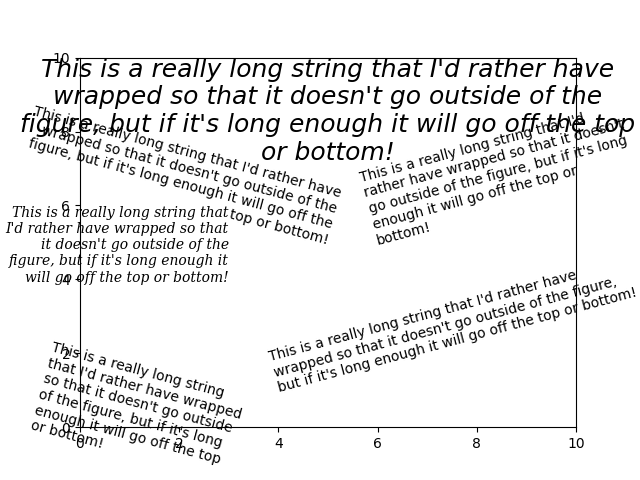# Auto-wrapping text#

Matplotlib can wrap text automatically, but if it's too long, the text will be displayed slightly outside of the boundaries of the axis anyways.

Note: Auto-wrapping does not work together with savefig(..., bbox_inches='tight'). The 'tight' setting rescales the canvas to accommodate all content and happens before wrapping. This affects %matplotlib inline in IPython and Jupyter notebooks where the inline setting uses bbox_inches='tight' by default when saving the image to embed.import matplotlib.pyplot as plt

fig = plt.figure()
plt.axis([0, 10, 0, 10])
t = ("This is a really long string that I'd rather have wrapped so that it "
"doesn't go outside of the figure, but if it's long enough it will go "
"off the top or bottom!")
plt.text(4, 1, t, ha='left', rotation=15, wrap=True)
plt.text(6, 5, t, ha='left', rotation=15, wrap=True)
plt.text(5, 5, t, ha='right', rotation=-15, wrap=True)
plt.text(5, 10, t, fontsize=18, style='oblique', ha='center',
va='top', wrap=True)
plt.text(3, 4, t, family='serif', style='italic', ha='right', wrap=True)
plt.text(-1, 0, t, ha='left', rotation=-15, wrap=True)

plt.show()


Keywords: matplotlib code example, codex, python plot, pyplot Gallery generated by Sphinx-Gallery